# Conic Sections

• Last Updated : 06 May, 2021

We know about basic curves such as a line and its various forms of equations. But every day we encounter different types of some too, for example – circle, parabola, hyperbola, etc. These curves are known as conic sections because they can be cut out from a cone. These curves have a very wide range of applications and are observed a lot in nature. Let’s see how to cut them out from a cone and what are their equations.

### Conic Sections

A Conic section, also referred just as a ‘Conic’ is a plane intersecting a cone. Imagine a cone being cut by a knife at different places creating different types of curves, which are known as Conic Sections. The three main Conic sections obtained are Parabola, Hyperbola, and ellipse (a Circle can be referred as a type of ellipse).

Attention reader! All those who say programming isn't for kids, just haven't met the right mentors yet. Join the  Demo Class for First Step to Coding Coursespecifically designed for students of class 8 to 12.

The students will get to learn more about the world of programming in these free classes which will definitely help them in making a wise career choice in the future.

Let’s say we take a fixed vertical line. We’ll call it “l”. Now make another line at a constant angle α from this line.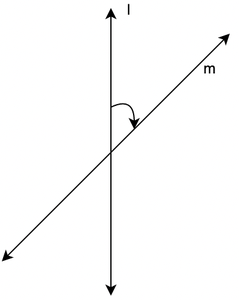Now if we start rotating the line m around l by keeping the angle the same. We will get a cone that extends to infinite in both directions.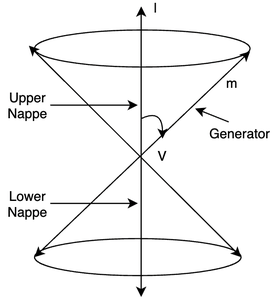The rotating line is called the generator of the cone. The vertical line is the axis of the cone. V is the vertex, it separates the cone into two parts called nappes.

Now when we take the intersection of the generated cone with a plane, the section obtained is called a conic section.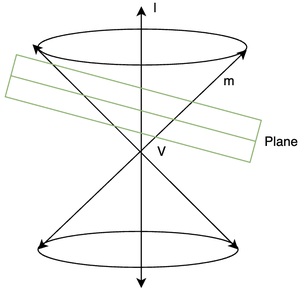This intersection generates different types of curves depending upon the angle of the plane that is intersecting with the cone.

### Generated Conic Sections

Depending upon the different angles at which the plane is intersecting the Cone, different types of curves are found. Imagine that an ice cream cone is in the hands, looking at the cone from the top of it will make it look like a circle because the top view of an inverted cone is a circle, that gives a conclusion that cutting a cone with a plane exactly at 90° will provide a circle, Similarly, different angles will lead to different types of curves.

Let’s see that plane makes an angle β with the vertical axis. Depending on the value of the angle there can be several curves of intersections.

1. If β = 90°. We get a circle.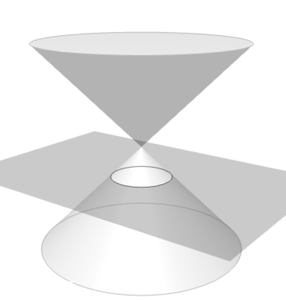2. If β lies between (α, 90°). We get an ellipse.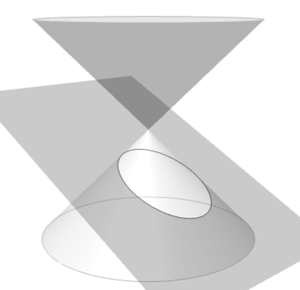3. If β = α. The generated shape is called a parabola.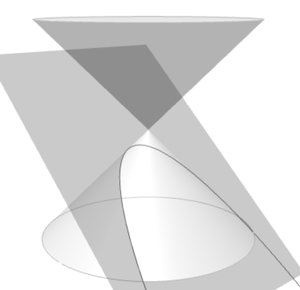4. If β lies in the interval [0, α]. The generated shape is called a hyperbola.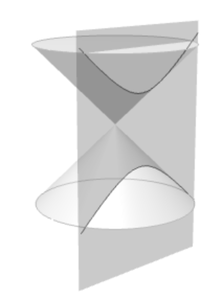### Circle

A circle is generated when the plane intersects the cone at right angles. As shown in the figure above, let’s look at it and define it in a more mathematical way,

A circle is a set of points which are equidistant from a fixed point. The fixed point is called centre of the circle and the distance is called radius.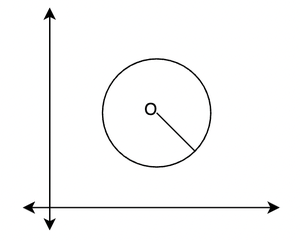O is the centre of the circle and the line joining the centre to the circle denotes the radius of the circle. Now let’s derive the equation for the circle.

Equation of the Circle

Let’s say the centre of the circle if given by C(h, k) and its radius is “r”. If we assume that P(x, y) is any point on the circle, then by the definition stated above, the distance of P from C must be “r”.This is the equation of the circle when the centre and the radius are given.

Let’s see some sample problems on these concepts.

### Sample Problems

Question 1: Find the equation of a circle that has a centre of (0,0) and a radius is 5.

Solution:

We have studied the formula for the equation of the circle.

(x-h)2 + (y – k)2 = r2

We just need to plug in the values in the formula.

Here, h = 0, k = 0 and r = 5

(x – 0)2 + (y – 0)2 = 52

⇒x2 + y2 = 52

⇒x2 + y2 = 25

Question 2: Find the equation of the circle with centre (-4, 5) and radius 4.

Solution:

The formula for the equation of the circle.

(x-h)2 + (y – k)2 = r2

We just need to plug in the values in the formula.

Here, h = -4, k = 5 and r = 4

(x – (-4))2 + (y – 5)2 = 52

⇒(x+ 4)2 + (y – 5)2 = 25

⇒x2 + 16 + 8x + y2 + 25 – 10y = 25

⇒x2 + 8x + y2 -10y + 16= 0

Question 3: The equation given below is an equation of the circle, find out the radius and the centre.

x2 + 6x + y2 – 4y = 3

Solution:

We are given the equation, now to find out the radius and the centre. We need to rearrange the equation such that this equation can come in the form given below.

(x-h)2 + (y – k)2 = r2

x2 + 6x + y2 – 4y = 3

⇒ x2 + (2)(3)x + y2 – 2(2)y = 3

We can see that these equations can be separated into two perfect squares.

⇒ x2 + (2)(3)x + 9 – 9 + y2 – 2(2)y + 4 – 4 = 3

⇒ (x + 3)2 – 9 + (y – 2)2 – 4 = 3

⇒ (x + 3)2 + (y – 2)2 = 3  + 4 + 9

⇒ (x + 3)2 + (y – 2)2 = 16

⇒ (x + 3)2 + (y – 2)2 = 42

Now comparing this equation with the standard equation of the circle, we notice,

h = -3, k = 2 and radius = 4.

Question 4: Find the equation of the circle, with centre (-h,-k) and radiusSolution:

The standard equation of the circle is given by,

(x-h)2 + (y – k)2 = r2

Here, we have h = -h and k = -k and radius =. Putting these values into the equation.Question 5: Let’s say we are given a line x + y = 2 and a circle that passes through the points (2,-2) and (3,4). It is also given that the centre of the circle lies on the line. Find out the radius and centre of the circle.

Solution:

Let’s say the equation of the circle is,

(x-h)2 + (y – k)2 = r2

Now we know that the centre of the circle lies on the line x + y = 2. Since the centre of the circle is (h, k), it should satisfy this line.

h + k = 2

Putting the value of h from this equation into the equation of the circle.

(x-(2 – k))2 + (y – k)2 = r2

Now we also know that the circle satisfies the points (2, -2) and (3,4). Putting (2,-2) in the above equation.

(2-(2 – k))2 + (-2 – k)2 = r2

⇒ k2 + (k + 2)2 = r2

⇒ k2 + k + 4 + 4k = r2

⇒ 2k2 + 4 + 4k = r2 …..(1)

Putting the equation (3,4) is,

(x-(2 – k))2 + (y – k)2 = r2

⇒ (3-(2 – k))2 + (4 – k)2 = r2

⇒(1 – k)2 + (4 – k)2 = r2

⇒ k2 -2k + 1 + 16 -8k + k2 =r2

⇒ 2k2 -10k + 17 =r2 ……(2)

Solving these equations we get,

h = 0.7, k = 1.7 and r2 = 12.58

My Personal Notes arrow_drop_up